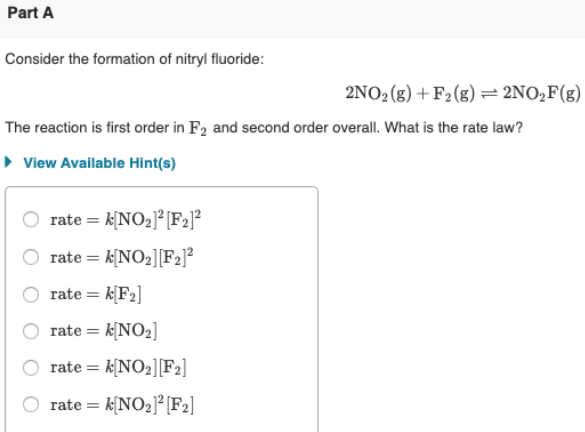# Consider the formation of nitryl fluoride: 2NO2 (g) + F2 (g) ⇌ 2NO2F(g) The reaction is first order in F2 and second order overall. What is the rate law? a) rate = k[NO2]^2[F2]^2 b) rate = k[NO2][F2]^2 c) rate = k[F2] d) rate = k[NO2] e) rate = k[NO2][F2] f) rate = k[NO2]^2[F2]